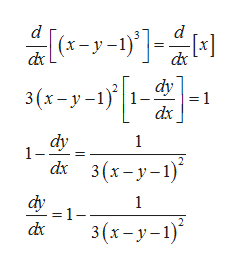# Find an equation of the tangent line to the graph of the function f defined by the following equation at the indicated point.(x − y − 1)3 = x; (1, −1)  y=

Question
88 views
Find an equation of the tangent line to the graph of the function f defined by the following equation at the indicated point.
(x − y − 1)3 = x; (1, −1)

y=
check_circle

star
star
star
star
star
1 Rating
Step 1

Given:

Step 2

Differentiate with respect to x as follows.help_outlineImage Transcriptionclose3 dc dy 1 =1 dx 3(х -у-1) dy 1- 1 3 (х-у-1)* dx 1 =1 3(х-у-1)° fullscreen
Step 3

At (1, –1), the deri...

### Want to see the full answer?

See Solution

#### Want to see this answer and more?

Solutions are written by subject experts who are available 24/7. Questions are typically answered within 1 hour.*

See Solution
*Response times may vary by subject and question.
Tagged in

### Derivative